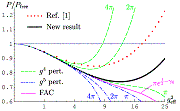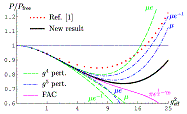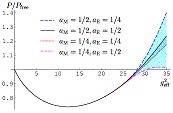[ Home ][ Data tables ]

# Andreas Ipp - Data tables## <->  Thermodynamics of large-Nf QCD at Finite Chemical Potential

 Authors: Andreas Ipp, Anton Rebhane-Print: hep-ph/0305030 Report-no: TUW-03-13 Abstract: We extend the previously obtained results for the thermodynamic potential of hot QCD in the limit of large number of fermions to non-vanishing chemical potential. [...]

Pressure at T=0
 Cutoff a=1/4 a=1/2 a=0.81 (P-P0)/Ng Data Data Data

(T=0, mubar=1, mu_q=1, g_eff^2=0 .. 40. First column is g_eff^2, second column is the pressure.)

1/2*Susceptibility at mu_q=0
 Cutoff a=1/4 a=1/2 (\chi-\chi0)/Ng/2 Data Data

(T=1, mubar=\pi T, mu_q=0, g_eff^2=0 .. 40. First column is g_eff^2, second column is the pressure. Data give the second derivative of the pressure with respect to mu_q. To get the susceptibility multiply by 2.)

1/12*Higher Susceptibility at mu_q=0
 Cutoff a=1/4 a=1/2 ((d^2/d\mu^2)\chi-\chi0))/Ng/12 Data Data

(T=1, mubar=\pi T, mu_q=0, g_eff^2=0 .. 40. First column is g_eff^2, second column is the pressure. Data give the forth derivative of the pressure with respect to mu_q. To get the higher susceptibility multiply by 12.)

Further data tables from the paper will be available soon.

## <->  Comment on and erratum to "Pressure of Hot QCD at Large Nf"

 Authors: Andreas Ipp, Guy D. Moore, Anton Rebhan Published in: Journal of High Energy Physics, JHEP 01 (2003) 037 e-Print: hep-ph/0301057 Report-no: TUW-02-26 Abstract: We repeat and correct the calculations of the thermodynamic potential of hot QCD in the limit of large number Nf of fermions. [...]

 PRESSURE Combined a=1/4 a=1/2 a=0.81 Graphs Complete result Data table Data Data Data GIF, EPS "nf"-part Minkowski (transverse) Data table Data Data Data GIF, EPS "nf"-part Minkowski (longitudinal) Data table Data Data Data GIF, EPS "1/2"-part Euclidean (transverse and longitudinal combined) Data table Data Data Data GIF, EPS Cutoff chosen Data table Data Data Data GIF, EPS

 LANDAU POLE and FAC Table Graphs Landau pole Data GIF, EPS FAC perturbation theory Data GIF, EPS

 GRAPHS FROM THE PAPER Comparison pi Comparison mu Cutoff ambiguityGIF, EPSGIF, EPSGIF, EPS

Tables: The data tables give the pressure over the free pressure. The first column is the effective coupling g_eff^2 from 0 to 35 in steps of 0.25. The second column gives the normalized pressure with different cutoffs. In the combined tables, the second column shows a=1/4, the third column a=1/2 and the last column a=0.81.

Graphs: The graphs show the combined plots for different cutoffs. In the pressure plots, green corresponds to a=1/4, blue to a=1/2, and black to a=0.81.

Computational details: For values of g_eff^2 > 22.5, the cutoff is chosen at Lambda_Landau / 2 (a=1/4), Lambda_Landau / sqrt(2) (a=1/2), and 0.9 Lambda_Landau (a=0.81). For smaller values of g_eff^2, a constant cutoff is chosen at 28.2, 39.9, and 50.7 (which is the cutoff for g_eff^2=22.5). This cutoff affects the spatial q-integration in Minkowski space, and the 4-dimensional Q-integration in Euclidean space. In Minkowski space omega is integrated up to 40 for all g_eff^2. The lower bounds for q and omega in Minkowski space are chosen at 10^-8, and the range closer than 10^-9 to the light-cone q=omega is taken out of Minkowski integration. The results given are accurate to at least 4 or 5 digits.Last update: Feb 19, 2003
Back to Andreas Ipp's Homepage.

Particle Physics Group TU Vienna

[ Home ][ Data tables ]